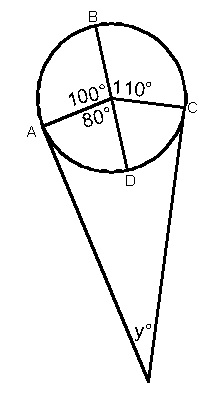# A geometry problem by A Former Brilliant Member

Geometry Level 1Two lines meet to form an angle of $y$ degrees and are tangent to a circle at points A and C, respectively. What is the value of $y$ in degrees?

×